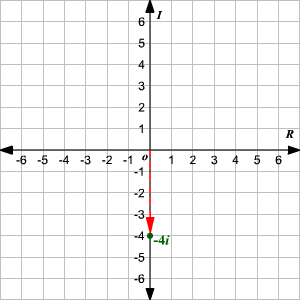# Complex Numbers

complex number  is a number of the form  $a+bi$ , where  $a$  and  $b$  are real numbers and  $i$  is the imaginary unit , the square root of $-1$ .

In a complex number  $z=a+bi$ , $a$ is called the "real part" of  $z$  and  $b$  is called the "imaginary part." If  $b=0$ , the complex number is a real number; if  $a=0$ , then the complex number is "purely imaginary."

We can graph a complex number on the Cartesian plane , using the horizontal axis as the real axis and the vertical axis as the imaginary axis. When we use the Cartesian plane this way, we call it the complex plane .

The complex number  $a+bi$ can be plotted as the ordered pair $\left(a,b\right)$ on the complex plane.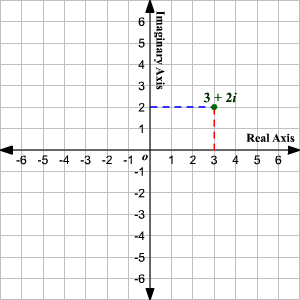The absolute value or modulus of a complex number $z=a+bi$ can be interpreted as the distance of the point $\left(a,b\right)$ from the origin on a complex plane.

Using the Distance Formula,

$\begin{array}{l}|z|=|a+bi|\\ \text{\hspace{0.17em}}\text{\hspace{0.17em}}\text{\hspace{0.17em}}\text{\hspace{0.17em}}\text{\hspace{0.17em}}=\sqrt{{\left(a-0\right)}^{2}+{\left(b-0\right)}^{2}}\\ \text{\hspace{0.17em}}\text{\hspace{0.17em}}\text{\hspace{0.17em}}\text{\hspace{0.17em}}\text{\hspace{0.17em}}=\sqrt{{a}^{2}+{b}^{2}}\end{array}$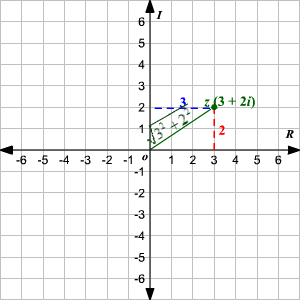Example 1:

Plot the number $-5+6i$ on a complex plane.

The real part of the complex number is $-5$ and the imaginary part is $6$ .

Start at the origin. Move $5$ units to the left on the real axis to reach the point $\left(-5,0\right)$ . Now, move $6$ units upward to reach the point $\left(-5,6\right)$ .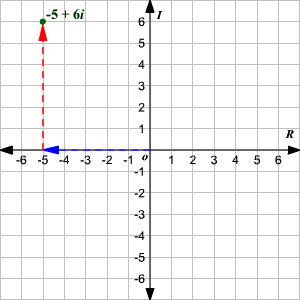If the real part of a complex number is zero, the number lies on the imaginary axis. Similarly, if the imaginary part of a complex number is zero, the number lies on the real axis.

Example 2:

Plot the number $6$ on the complex plane.

The real part of the complex number is $6$ and the imaginary part is $0$ . So, the number will lie on the real axis.

Start at the origin. Move $6$ units to the right on the real axis to reach the point $\left(6,0\right)$ .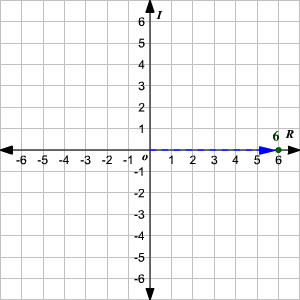Example 3:

Plot the number $-4i$ on the complex plane.

The real part of the complex number $-4i$ is zero and the imaginary part is $-4$ .

Start at the origin. Move $4$ units down along the imaginary axis to reach the point $\left(0,-4\right)$ .